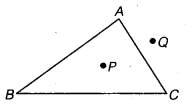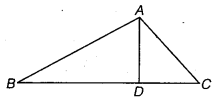# Class 6 Maths NCERT Solutions for Chapter 4 Basic Geometrical Ideas Ex – 4.4

## Basic Geometrical Ideas

Question 1.
Draw a rough sketch of a triangle ABC. Mark a point P in its interior and a point Q in its exterior. Is the point A in its exterior or in its interior?

Solution:
Rough sketch of a ∠ABC as shown.Points P and Q are marked in the interior and exterior of ∆ABC.
Point A lies on the ∆ABC.

Question 2.
(a) Identify three triangles in the figure.
(b) Write the names of seven angles.
(c) Write the names of six line segments.
(d) Which two triangles have ZB as common?Solution:
(a)
Three triangles are identified in the figure as ∆ ABC, ∆ ABD and ∆ ACD.